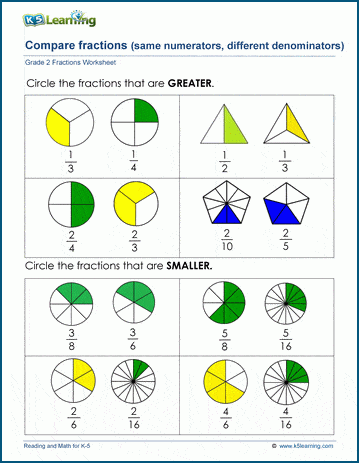# Comparing fractions

## Comparing fractions worksheets: same numerators

In these worksheets, students compare fractions with the same numerator and different denominators.  All fractions are illustrated by pictured shapes divided into equal parts.  Students will learn that, given the same numerator, the shape with the smaller denominator will be larger.## More fractions worksheets

Explore all of our fractions worksheets, from dividing shapes into "equal parts" to multiplying and dividing improper fractions and mixed numbers.

## What is K5?

K5 Learning offers reading and math worksheets, workbooks and an online reading and math program for kids in kindergarten to grade 5.  We help your children build good study habits and excel in school.